# Processing Sound Ranges part 3 Barb Ericson Georgia

• Slides: 22Processing Sound Ranges part 3 Barb Ericson Georgia Institute of Technology Oct 2009 Using. Sound. Ranges-part 3 1Learning Goals • Processing ranges of Sound values – Creating echoes – Changing the sound frequency • Computing concepts – Changing a for loop to increment by a value other than 1 – Modifying a method to be more general Using. Sound. Ranges-part 3 2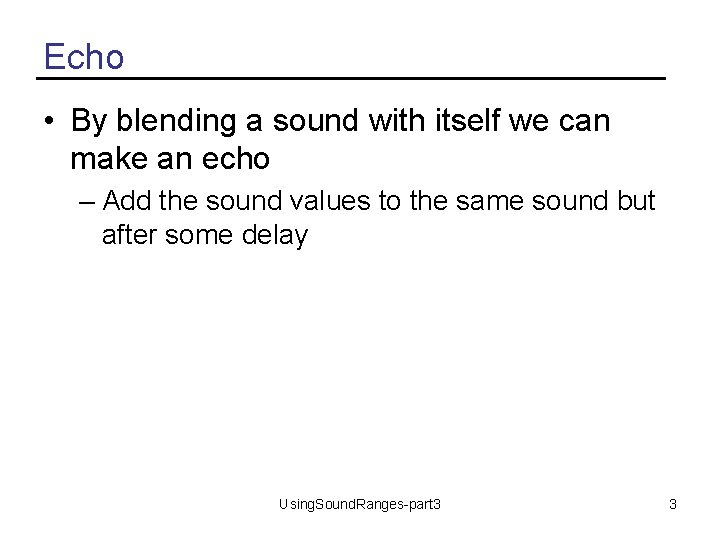Echo • By blending a sound with itself we can make an echo – Add the sound values to the same sound but after some delay Using. Sound. Ranges-part 3 3Method echo public void echo(int delay) { // make a copy of the original sound Sound s = new Sound(this. get. File. Name()); int value = 0; // loop from delay to end of sound for (int i = delay; i < this. get. Length(); i++) { Using. Sound. Ranges-part 3 4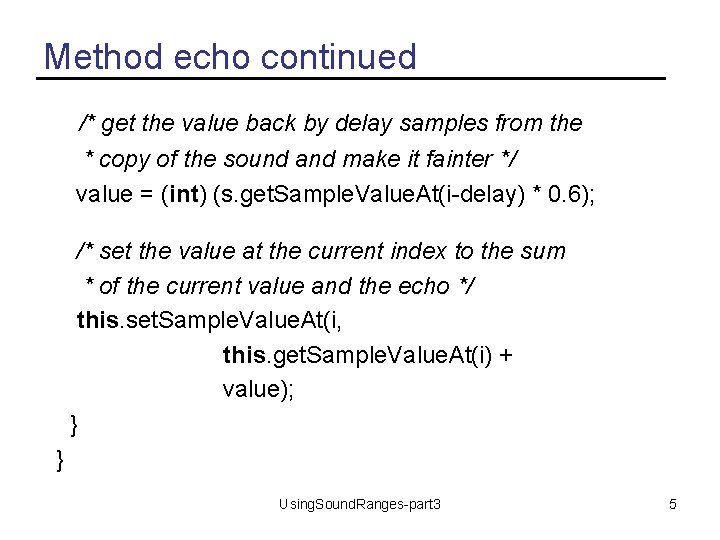Method echo continued /* get the value back by delay samples from the * copy of the sound and make it fainter */ value = (int) (s. get. Sample. Value. At(i-delay) * 0. 6); /* set the value at the current index to the sum * of the current value and the echo */ this. set. Sample. Value. At(i, this. get. Sample. Value. At(i) + value); } } Using. Sound. Ranges-part 3 5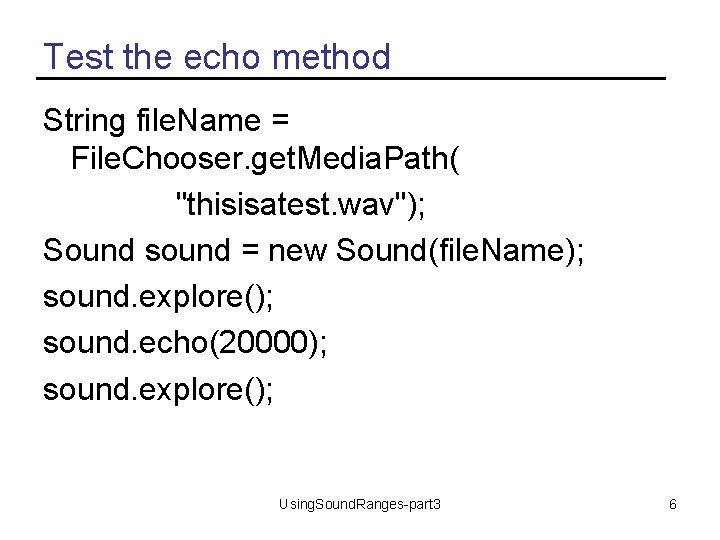Test the echo method String file. Name = File. Chooser. get. Media. Path( "thisisatest. wav"); Sound sound = new Sound(file. Name); sound. explore(); sound. echo(20000); sound. explore(); Using. Sound. Ranges-part 3 6How does that work? • The echo method takes an integer delay – The number of samples to wait before starting the echo • It makes a copy of the current sound – So that we copy without the echo • Loop starting from delay to the end of the sound – Get the value at i-delay and multiply it by 0. 6 to make it quieter – Set the current sound value at i to the current value at i plus the calculated value Using. Sound. Ranges-part 3 7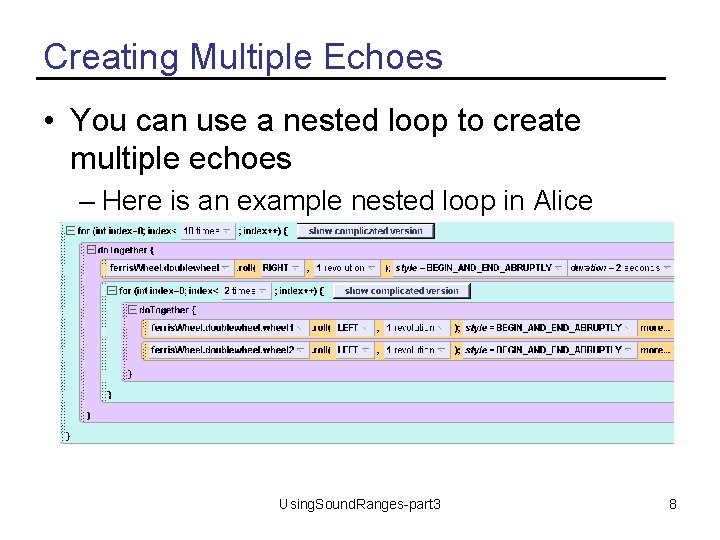Creating Multiple Echoes • You can use a nested loop to create multiple echoes – Here is an example nested loop in Alice Using. Sound. Ranges-part 3 8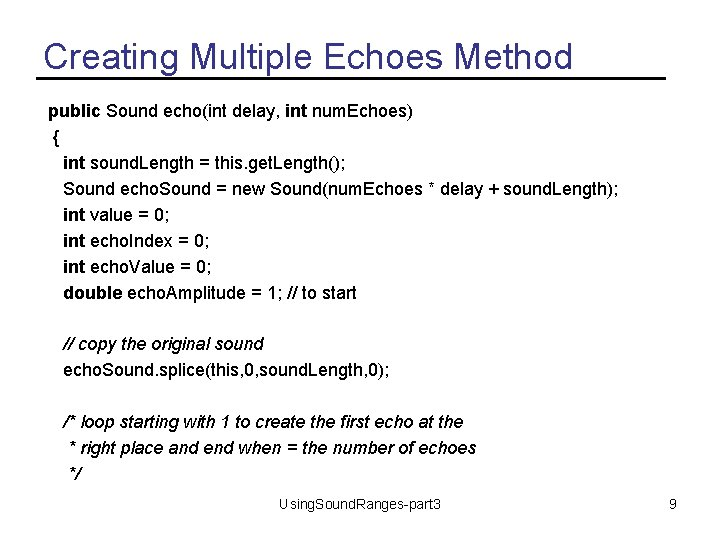Creating Multiple Echoes Method public Sound echo(int delay, int num. Echoes) { int sound. Length = this. get. Length(); Sound echo. Sound = new Sound(num. Echoes * delay + sound. Length); int value = 0; int echo. Index = 0; int echo. Value = 0; double echo. Amplitude = 1; // to start // copy the original sound echo. Sound. splice(this, 0, sound. Length, 0); /* loop starting with 1 to create the first echo at the * right place and end when = the number of echoes */ Using. Sound. Ranges-part 3 9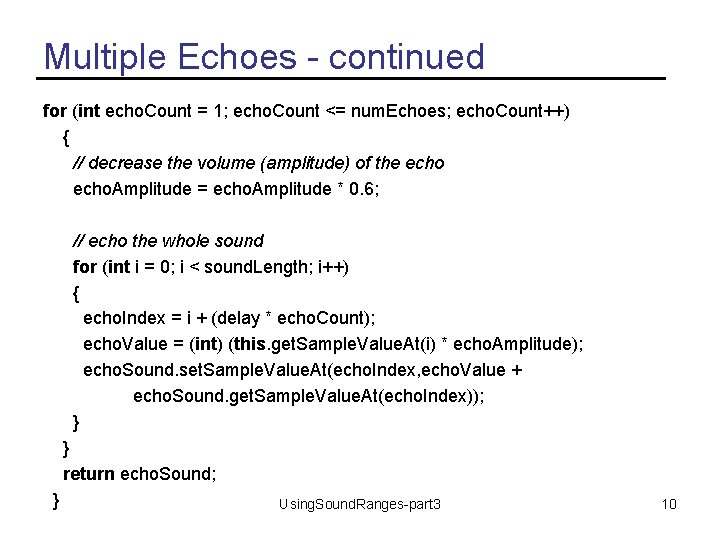Multiple Echoes - continued for (int echo. Count = 1; echo. Count <= num. Echoes; echo. Count++) { // decrease the volume (amplitude) of the echo. Amplitude = echo. Amplitude * 0. 6; // echo the whole sound for (int i = 0; i < sound. Length; i++) { echo. Index = i + (delay * echo. Count); echo. Value = (int) (this. get. Sample. Value. At(i) * echo. Amplitude); echo. Sound. set. Sample. Value. At(echo. Index, echo. Value + echo. Sound. get. Sample. Value. At(echo. Index)); } } return echo. Sound; } Using. Sound. Ranges-part 3 10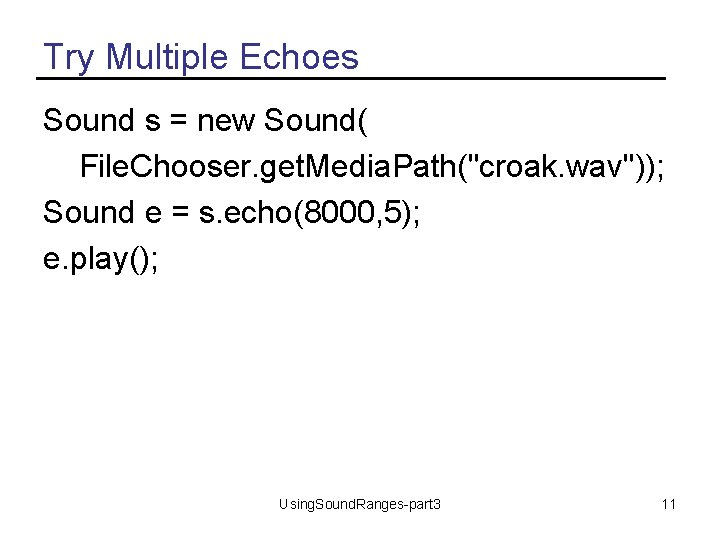Try Multiple Echoes Sound s = new Sound( File. Chooser. get. Media. Path("croak. wav")); Sound e = s. echo(8000, 5); e. play(); Using. Sound. Ranges-part 3 11Changing the Sound Frequency • The frequency of a wave is the number of cycles per second (cps), or Hertz (Hz) – (Complex sounds have more than one frequency in them. ) • Our perception of pitch is related (logarithmically) to changes in frequency – Higher frequencies are perceived as higher pitches – We can hear between 5 Hz and 20, 000 Hz (20 k. Hz) – A above middle C is 440 Hz Using. Sound. Ranges-part 3 12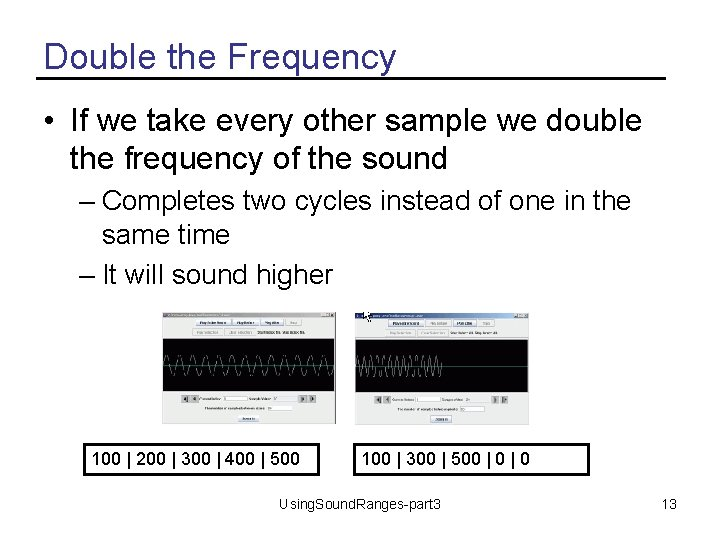Double the Frequency • If we take every other sample we double the frequency of the sound – Completes two cycles instead of one in the same time – It will sound higher 100 | 200 | 300 | 400 | 500 100 | 300 | 500 | 0 Using. Sound. Ranges-part 3 13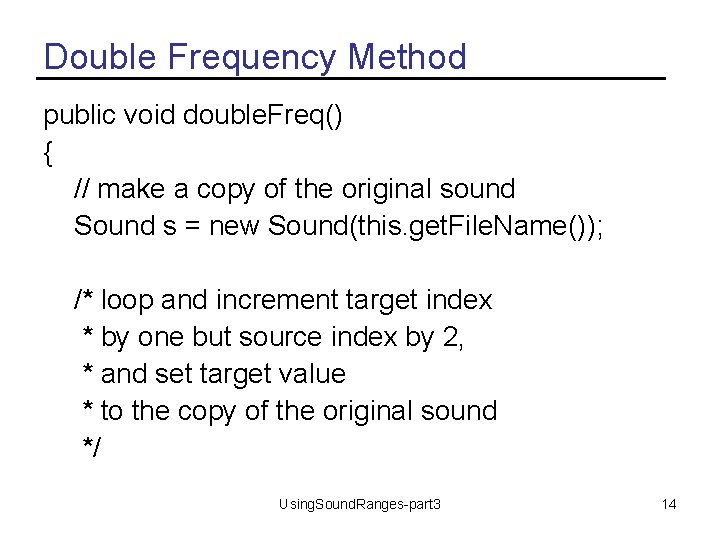Double Frequency Method public void double. Freq() { // make a copy of the original sound Sound s = new Sound(this. get. File. Name()); /* loop and increment target index * by one but source index by 2, * and set target value * to the copy of the original sound */ Using. Sound. Ranges-part 3 14Double Frequency - Continued for (int source. Index=0, target. Index = 0; source. Index < this. get. Length(); source. Index=source. Index+2, target. Index++) this. set. Sample. Value. At(target. Index, s. get. Sample. Value. At(source. Index)); // clear out the rest of this sound to silence (0) for (int i = this. get. Length() / 2; i < this. get. Length(); i++) this. set. Sample. Value. At(i, 0); } Using. Sound. Ranges-part 3 15Test Double Frequency Sound s = new Sound(File. Chooser. get. Media. Path( "c 4. wav")); s. explore(); s. double. Freq(); s. explore(); Using. Sound. Ranges-part 3 16Challenge • Create a method that will take every third sample – Will this sound higher or lower? • Can you make this method more general? – By passing in the amount to add to the source index each time? Using. Sound. Ranges-part 3 17Halving the Frequency • We can copy each source value twice to half the frequency – Only get through half a cycle in the same time we used to get through a full cycle – It will sound lower 100 | 200 | 300 | 400 | 500 100 | 200 | 300 Using. Sound. Ranges-part 3 18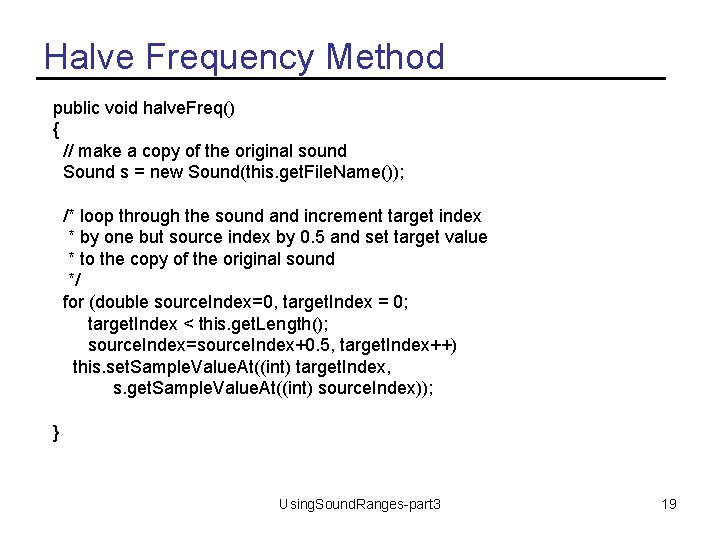Halve Frequency Method public void halve. Freq() { // make a copy of the original sound Sound s = new Sound(this. get. File. Name()); /* loop through the sound and increment target index * by one but source index by 0. 5 and set target value * to the copy of the original sound */ for (double source. Index=0, target. Index = 0; target. Index < this. get. Length(); source. Index=source. Index+0. 5, target. Index++) this. set. Sample. Value. At((int) target. Index, s. get. Sample. Value. At((int) source. Index)); } Using. Sound. Ranges-part 3 19Testing Halve Frequency Sound s = new Sound(File. Chooser. get. Media. Path( "c 4. wav")); s. explore(); s. halve. Freq(); s. explore(); Using. Sound. Ranges-part 3 20Challenge • Write a method that will copy each sound value 4 times to the target – Will the new sound be higher or lower? • Can you make this more general? – By passing in the number of times to copy the source value – Try it with 3 times and check the index values to make sure that you are doing it right Using. Sound. Ranges-part 3 21Summary • You can increment or decrement loop variables by numbers other than 1 • You can make methods more general – By passing in parameters Using. Sound. Ranges-part 3 22